085-1263404

### My Journal

All things Mathematical
20 Jun 2018
##### Question 1

A particle moves along a straight line and its distance from a fixed point in the straight line is given by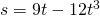. Calculate its average speed in the interval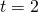to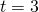; its instantaneous speed at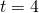. Find when and where its instantaneous speed is zero. Describe in a general way the motion of the particle.

##### Question 2 (a)

A particle is projected at angle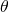to the horizontal up an inclined plane of inclination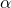. Show that is hodograph is represented by a vertical line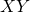where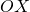is its initial speed, and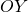its speed on striking the plane. Show that if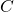is the middle point ofthat the angle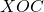is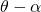and that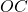multiplied by the time of flight is equal to the range on the inclined plane.

OR

##### Question 2 (b)

A particle is projected at an inclinationwith a speed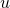sufficient to enable it to strike a point on a wall which is at a horizontal distance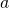from the point of projection. Find the height of the point on the wall struck by the particle in terms of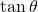. Hence find an expression for the maximum height on the wall which can be reached by the particle.

##### Question 3

A trolley is pulled along a horizontal table by a string passing over a pulley and carrying a scale pan and weights so that the total load on the string is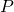grams. The acceleration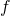in cm. per sec. per sec. for different values ofis given in the following table :-…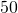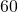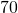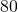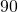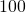…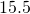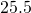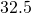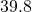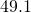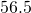Explain how you would treat these observations.

(1) to obtain the value ofrequired to make the system move with uniform speed.

(2) to exemplify the second law of motion.

(3) to deduce approximately the mass of the trolley.

##### Question 4

Why is the outer rail of a railway track raised on a curve? If the gauge is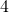ft.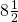in. and the radius of the curve is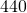yds., find how much the outer rail must be raised for an engine going round the curve at 30 miles an hour. [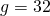ft./sec]

##### Question 5

A nail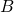is driven into a wall vertically below a point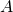to which is attached a pendulum bob by a string of length(being greater than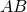). The bob is raised to the level ofwith the string taut and then released. The string strikes the nail at, and the bob just makes one complete revolution about. Find the value ofin terms of.

##### Question 6

An oil-electric coach weights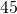tons when fully loaded and is equipped with an engine of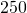h.p. Taking the resistance to motion on the level as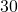lb. per ton; find the speeds which can be attained (1) on the horizontal, (2) up an incline with a gradient ofin.

##### Question 7

A regular hexagon is constructed of rods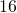in. long, each weighing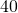grams. Each corner is loaded in the following order going round the hexagon with,,,,andgrams, respectively. Find the centre of gravity of the whole.

##### Question 8

The beam of a balance weighs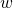grams and its centre of gravity is at a distance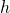cm. below the central knife-edge. When the beam is in equilibrium with the scale pans removed, the outer knife-edges, from which hand the scale-pans, are at a horizontal distancefrom the central knife-edge and each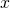cm. higher than the central knife-edge. If weights of the scale-pans and their loads are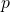and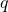respectively, find an expression for the inclination of the beam to the horizontal.

##### Question 9

A string of length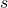is fastened to the ends of a uniform rod of length. Using a suitable length of string it is possible to find a point on it at a distancefrom one end so that if the rod is suspended by holding the string at the point the portionis horizontal. Find an equation forand show that the length of the string should not exceed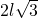.

##### Question 10

A body of weightis dragged with uniform speed up a plane inclined at an angleto the horizontal by a forceapplied to it in a direction inclined to the plane at an angle. Assuming the ordinary laws of friction, determine the magnitude of the forceand the work done dragging the body up the plane.

##### Citation:

State Examinations Commission (2018). State Examination Commission. Accessed at: https://www.examinations.ie/?l=en&mc=au&sc=ru

Malone, D and Murray, H. (2016). Archive of Maths State Exams Papers. Accessed at: http://archive.maths.nuim.ie/staff/dmalone/StateExamPapers/

##### Licence:

“Contains Irish Public Sector Information licensed under a Creative Commons Attribution 4.0 International (CC BY 4.0) licence”.

The EU Directive 2003/98/EC on the re-use of public sector information, its amendment EU Directive 2013/37/EC, its transposed Irish Statutory Instruments S.I. No. 279/2005, S.I No. 103/2008, and S.I. No. 525/2015, and related Circulars issued by the Department of Finance (Circular 32/05), and Department of Public Expenditure and Reform (Circular 16/15 and Circular 12/16).

Note. Circular 12/2016: Licence for Re-Use of Public Sector Information adopts CC-BY as the standard PSI licence, and notes that the open standard licence identified in this Circular supersedes PSI General Licence No: 2005/08/01.